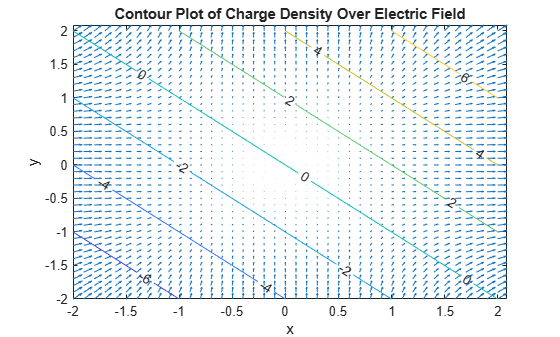Main Content

# divergence

Divergence of vector field

## Syntax

``divergence(V,X)``

## Description

example

````divergence(V,X)` returns the divergence of vector field `V` with respect to the vector `X` in Cartesian coordinates. Vectors `V` and `X` must have the same length.```

## Examples

collapse all

Find the divergence of the vector field V(x,y,z) = (x, 2y2, 3z3) with respect to vector X = (x,y,z).

```syms x y z field = [x 2*y^2 3*z^3]; vars = [x y z]; divergence(field,vars)```
```ans = 9*z^2 + 4*y + 1```

Show that the divergence of the curl of the vector field is 0.

`divergence(curl(field,vars),vars)`
```ans = 0```

Find the divergence of the gradient of this scalar function. The result is the Laplacian of the scalar function.

```syms x y z f = x^2 + y^2 + z^2; divergence(gradient(f,vars),vars)```
```ans = 6```

Gauss’ Law in differential form states that the divergence of electric field is proportional to the electric charge density.

`$\underset{}{\overset{\to }{\nabla }}\cdot \underset{}{\overset{\to }{E}}\left(\underset{}{\overset{\to }{r}}\right)=\frac{\rho \left(\underset{}{\overset{\to }{r}}\right)}{{ϵ}_{0}}\phantom{\rule{0.2777777777777778em}{0ex}}.$`

Find the electric charge density for the electric field $\underset{}{\overset{\to }{E}}={x}^{2}\underset{}{\overset{ˆ}{i}}+{y}^{2}\underset{}{\overset{ˆ}{j}}$.

```syms x y ep0 E = [x^2 y^2]; rho = divergence(E,[x y])*ep0```
`rho = ${\mathrm{ep}}_{0} \left(2 x+2 y\right)$`

Visualize the electric field and electric charge density for `-2 < x < 2` and `-2 < y < 2` with `ep0 = 1`. Create a grid of values of `x` and `y` using `meshgrid`. Find the values of electric field and charge density by substituting grid values using `subs`. Simultaneously substitute the grid values `xPlot` and `yPlot` into the charge density `rho` by using cells arrays as inputs to `subs`.

```rho = subs(rho,ep0,1); v = -2:0.1:2; [xPlot,yPlot] = meshgrid(v); Ex = subs(E(1),x,xPlot); Ey = subs(E(2),y,yPlot); rhoPlot = double(subs(rho,{x,y},{xPlot,yPlot}));```

Plot the electric field using `quiver`. Overlay the charge density using `contour`. The contour lines indicate the values of the charge density.

```quiver(xPlot,yPlot,Ex,Ey) hold on contour(xPlot,yPlot,rhoPlot,'ShowText','on') title('Contour Plot of Charge Density Over Electric Field') xlabel('x') ylabel('y')```## Input Arguments

collapse all

Vector field to find divergence of, specified as a symbolic expression or function, or as a vector of symbolic expressions or functions. `V` must be the same length as `X`.

Variables with respect to which you find the divergence, specified as a symbolic variable or a vector of symbolic variables. `X` must be the same length as `V`.

## More About

collapse all

### Divergence of Vector Field

The divergence of the vector field V = (V1,...,Vn) with respect to the vector X = (X1,...,Xn) in Cartesian coordinates is the sum of partial derivatives of V with respect to X1,...,Xn.

`$div\left(\stackrel{\to }{V}\right)=\nabla \cdot \stackrel{\to }{V}=\sum _{i=1}^{n}\frac{\partial {V}_{i}}{\partial {x}_{i}}.$`

## See Also

Introduced in R2012a

## Support

#### Mathematical Modeling with Symbolic Math Toolbox

Get examples and videos